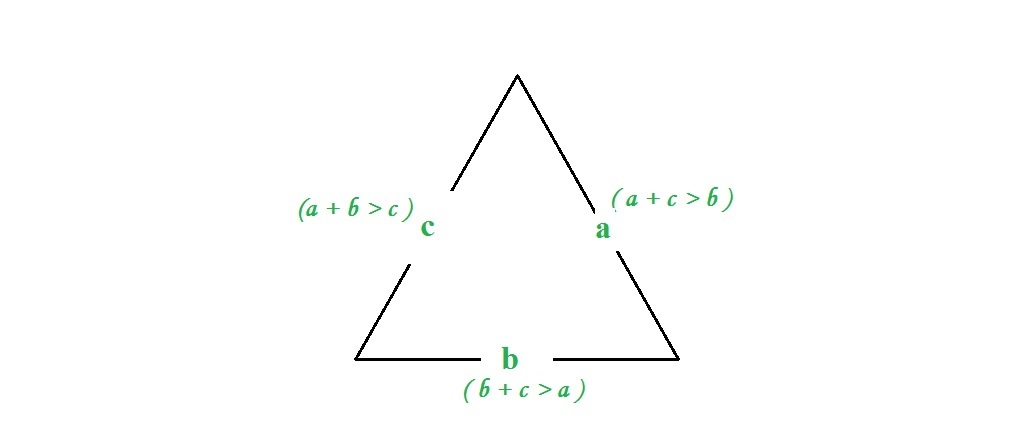# Python | Checking triangular inequality on list of lists

Given a list of lists, the task is to find whether a sublist satisfies the triangle inequality.

The triangle inequality states that for any triangle, the sum of the lengths of any two sides must be greater than or equal to the length of the remaining side. In other words, a triangle is valid if sum of its two sides is greater than the third side. If three sides are a, b and c, then three conditions should be met.

```a + b > c
a + c > b
b + c > a
```Method #1 : Using List comprehension

 `# Python code to find whether a sublist  ` `# satisfies the triangle inequality. ` ` `  `# List initialization ` `Input` `=` `[[``1``, ``3``, ``1``], [``4``, ``5``, ``6``]] ` ` `  `# Sorting sublist ` `for` `elem ``in` `Input``: ` `    ``elem.sort() ` ` `  `# Using list comprehension ` `Output ``=` `[(p, q, r) ``for` `p, q, r ``in` `Input` `if` `(p ``+` `q)>``=` `r] ` ` `  `# Printing output ` `print``(Output) `

Output:

```[(4, 5, 6)]
```

Method #2 : Using Iteration

 `# Python code to find whether a sublist ` `# satisfies the triangle inequality. ` ` `  `# List initialization ` `Input` `=` `[[``1``, ``1``, ``3``], [``4``, ``5``, ``6``]] ` ` `  `# Sorting sublist of list of list ` `for` `elem ``in` `Input``: ` `    ``elem.sort() ` ` `  `# Checking for triangular inequality ` `for` `elem ``in` `Input``: ` `    ``if` `elem[``0``] ``+` `elem[``1``] > elem[``2``]: ` `        ``print``(elem) `

Output:

```[4, 5, 6]
```

My Personal Notes arrow_drop_upIf you like GeeksforGeeks and would like to contribute, you can also write an article using contribute.geeksforgeeks.org or mail your article to contribute@geeksforgeeks.org. See your article appearing on the GeeksforGeeks main page and help other Geeks.

Please Improve this article if you find anything incorrect by clicking on the "Improve Article" button below.

Article Tags :

Be the First to upvote.

Please write to us at contribute@geeksforgeeks.org to report any issue with the above content.